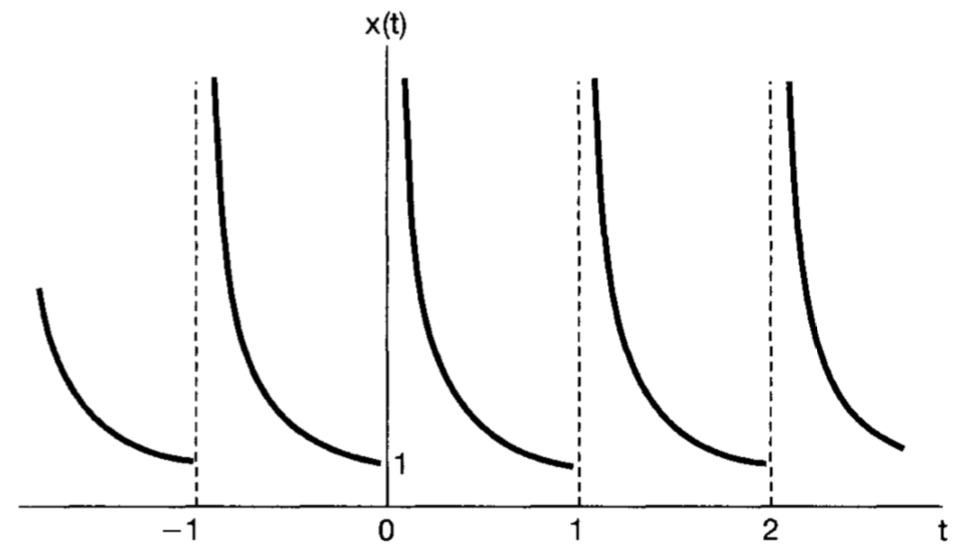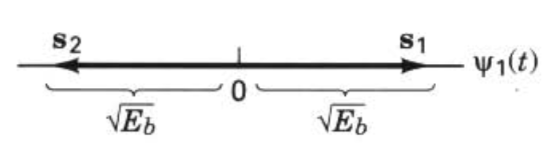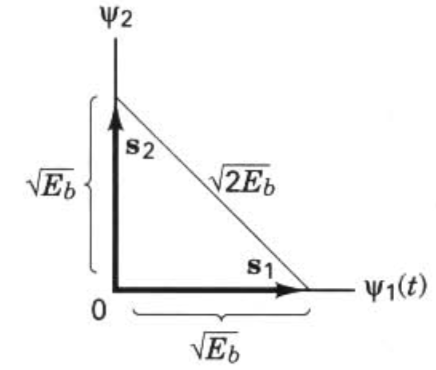## Signals and Systems

### Function transformations:

#### h(t) to other forms:

Before making convolution in time domain, we need to transform impulse response function into the required format:
Example 1:
We have $$h(t)=s(T-t)$$.
We will make convolution as $$z(t)=r(t)*h(t)=\int_0^t r(\tau)h(t-\tau)d\tau$$.
Impulse response should pass these stages:
$$h(t)=s(T-t)$$
$$h(\tau)=s(T-\tau)$$
$$h(-\tau)=s(-T+\tau)$$
$$h(t-\tau)=s(t-T+\tau)$$
Substitute into $$\int_0^t r(\tau)h(t-\tau)d\tau=\int_0^t r(\tau)s(t-T+\tau)d\tau$$

Example 2:
We have $$h(t)=u(t)$$.
We will make convolution as $$\int_0^t x(\tau)h(t-\tau)d\tau$$.
$$h(t)=u(t)$$
$$h(\tau)=u(\tau)$$
$$h(-\tau)=u(-\tau)$$
$$h(t-\tau)=u(t-\tau)$$
Then substitute.

### Convergence of the Fourier Series

Periodic signals can be represented by Fourier series under certain conditions:

1. Energy of the signal over one period should be finite.
For example, the signal violates this condition:
$$x(t)=\frac{1}{t}$$, Period: $$0< t\leq1$$2. Signal must have finite number of maxima and minima in a finite time interval.
3. Signal must have finite number of discontinuity in a finite time interval.

## Telecommunications

### Error Probability Performance

Bit error probability of equally likely, binary signals:
$$P_B=\int_{\frac{a_1-a_2}{2\sigma_0}}^\infty \frac{1}{\sqrt{2\pi}}e^{-\frac{1}{2}(u)^2}du=Q(\frac{a_1-a_2}{2\sigma_0})$$
In the nominator of argument of $$Q$$, we see voltage difference (square root of power or square root of energy) of signals.
$$p^2=\frac{v^2}{R}$$, $$power=\frac{Energy}{time}$$
$$[\frac{volt^2}{ohm}]=[watt]=[\frac{Joule}{sec}]$$
$$[\frac{volt}{ohm}]=[\frac{Coulomb}{sec}] \Longrightarrow [\frac{volt*sec}{ohm}]=[Coulomb] \Longrightarrow [\frac{volt^2*sec}{ohm}]=[volt*Coulomb]=[Joule]$$
Taking the square root of Joule gives not only volt but $$[\frac{volt*\sqrt{sec}}{\sqrt{ohm}}]$$. Since in the questions, both transmitted signals and noise values are given, the $$sec$$s (maybe $$ohm$$s (?)) cancel each other and no need to consider.

The nominator is the difference between signals in terms of voltage.

1. For antipodal signals, the difference is $$2*\sqrt{E_b}$$. For $$s_1(t)$$, $$a_1=\sqrt{E_b}$$.
For $$s_1(t)$$, $$a_2=-\sqrt{E_b}$$.
$$a_1-a_2=2*\sqrt{E_b}$$.
$$E_b$$: energy of a bit

The variance of noise is given as $$\frac{N_0}{2}$$ in general.

$$Q(\frac{a_1-a_2}{2\sigma_0})=Q(\frac{1}{2}\frac{2*\sqrt{E_b}}{\sqrt{\frac{N_0}{2}}})=Q(\sqrt{\frac{2E_b}{N_0}})$$

Or equivalently, $$Q(\frac{a_1-a_2}{2\sigma_0})=Q(\frac{1}{2}\frac{\sqrt{E_d}}{\sqrt{\frac{N_0}{2}}})=Q(\sqrt{\frac{E_d}{2N_0}})$$2. For orthogal signals, the difference is $$\sqrt{2*E_b}$$.
$$Q(\frac{a_1-a_2}{2\sigma_0})=Q(\frac{1}{2}\frac{\sqrt{2E_b}}{\sqrt{\frac{N_0}{2}}})=Q(\sqrt{\frac{E_b}{N_0}})$$